### SOLUTIONS TO INTEGRATION OF EXPONENTIAL FUNCTIONS

SOLUTION 6 : Integrate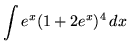. Use u-substitution. Let

u = 1+2ex

so that

du = 2ex dx ,

or

(1/2) du = ex dx .

Substitute into the original problem, replacing all forms of x, getting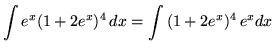.

SOLUTION 7 : Integrate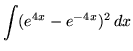. First, square the exponential function, recalling that (A-B)2 = A2 - 2AB + B2 . The result is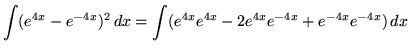(Recall that.)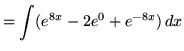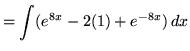(Use the properties of integrals.)(Use formula 3 from the introduction to this section on integrating exponential functions.).

SOLUTION 8 : Integrate. Use u-substitution. Let

u = 1+ex

so that

du = ex dx .

In addition, we can "back substitute" with

ex = u-1 .

Substitute into the original problem, replacing all forms of x, getting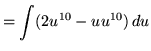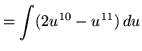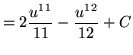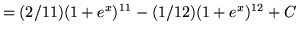.

SOLUTION 9 :Integrate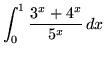. First, split the function into two parts, getting(Recall that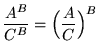.)(Use formula 2 from the introduction to this section on integrating exponential functions.)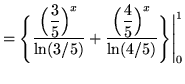.